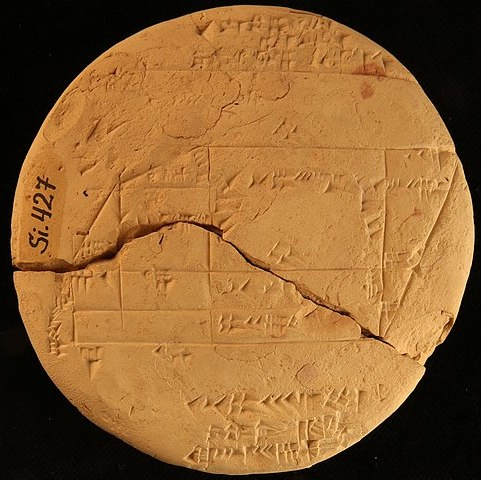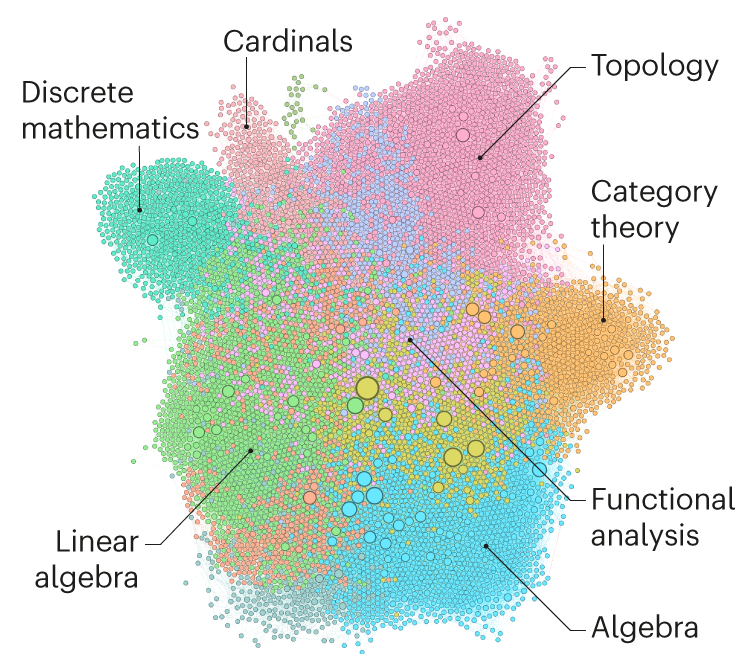# Tony’s Take August 2021

## This month’s topics:

### Old Babylonian Applied Geometry

The story was picked up by The Guardian, the Smithsonian’s SmartNews, Science News, Arab News and Popular Mechanics. Donna Lu’s coverage in The Guardian (August 4, 2021) has the headline “Australian mathematician discovers applied geometry engraved on 3,700-year-old tablet”, with subhead “Old Babylonian tablet likely used for surveying uses Pythagorean triples at least 1,000 years before Pythagoras.”

Lu begins: “Known as Si.427, the tablet bears a field plan measuring the boundaries of some land. The tablet dates from the Old Babylonian period between 1900 and 1600 BCE and was discovered in the late 19th century in what is now Iraq. It had been housed in the Istanbul Archaeological Museum before Dr Daniel Mansfield from the University of New South Wales tracked it down.”Obverse of the tablet Si.427, diameter 10cm, in a photograph, fromthe Istanbul Archaeological Museum, courtesy of Daniel Mansfield.

First note that the area surveyed in the tablet has been partitioned into simple geometrical shapes (rectangles, right triangles, trapezoids) for which the Babylonians knew how to calculate the area from the linear dimensions.Part of the transliteration of Si.427, adapted from Mansfield’s article in Journal of Cuneiform Studies. Mansfield has added labels for the vertices, [replacements of obliterated data] (explanatory interpolations), and ; to mark off the units in the base-60 numbers.Red: lengths in rods. Blue: areas in sar, iku, eše.For example, the rectangle $ABIH$ has sides marked 50 and 22;40 (rods; a Babylonian nindam, or rod, is about 20 feet) and area given as 1 (eše) 5 (iku) and 33$\frac{1}{3}$ sar. The sar is the unit of area corresponding to 1 square rod; an iku is 100 sar (about an acre) and an eše is 600 sar. In Babylonian base-60 notation, 22;40 = 22$\frac{2}{3}$; and in fact $50\times 22\frac{2}{3} = 1133\frac{1}{3}$ square rods (i.e. sar) $=$ 1 eše 5 iku and 33$\frac{1}{3}$ sar, as indicated. Similarly the right trapezoid $JFKO$ has height 7;30 = $7\frac{1}{2}$ and bases $6;10+18+50+6;40 = 80;50$ and $6;10+18+50+5 = 79;10$ with average 80, so its area is $80 \times 7\frac{1}{2} = 600$ sar, or 1 eše, as shown.

Mansfield noticed the presence of two or maybe three Pythagorean triangles (right triangles with whole-number sides) in the diagram, including the triangle $ADE$ with sides $7;30$ and 18 ($\frac{3}{2}$ of the 5, 12, 13 right triangle) and the rectangle $GHML$, equal to two copies of $ADE$. He argues that measuring out Pythagorean triangles in the terrain (a practice still in use today) was a way to guarantee right angles and accurate area computations.

### Computer assists lofty theory

Davide Castelvecchi’s contribution to Nature for June 18, 2021 was “Mathematicians welcome computer-assisted proof in ‘grand unification’ theory,” with sub-head “Proof-assistant software handles an abstract concept at the cutting edge of research, revealing a bigger role for software in mathematics.” He tells us how Peter Scholze (Bonn; “considered one of mathematics’ brightest stars and has a track record of introducing revolutionary concepts”) and his collaborator Dustin Clausen (Copenhagen) have set forth an “ambitious plan,” which they call condensed mathematics: “they say it promises to bring new insights and connections between fields ranging from geometry to number theory. … Until now, much of that vision rested on a technical proof so involved that even Scholze and Clausen couldn’t be sure it was correct. But earlier this month, Scholze announced that a project to check the heart of the proof using specialized computer software had been successful.”

The specialized software in question is a proof assistant. Castelvecchi: “Proof assistants … force the user to lay out the logic of their arguments in a rigorous way, and they fill in simpler steps that human mathematicians had consciously or unconsciously skipped. … In this way, proof assistants can help to verify mathematical proofs that would otherwise be time-consuming and difficult, perhaps even practically impossible, for a human to check.”

Castelvecchi goes on to tell us something about condensed mathematics, and the ensuing ‘grand unification’ project; finally: “Around 2018, Scholze and Clausen began to realize that the conventional approach to the concept of topology led to incompatibilities between [the] three mathematical universes — geometry, functional analysis and $p$-adic numbers — but that alternative foundations could bridge those gaps.”

“There was one catch, however: to show that geometry fits into this picture, Scholze and Clausen had to prove one highly technical theorem about the set of ordinary real numbers.” Scholze found a proof, but is was so novel and complex “that Scholze himself worried there could be some subtle gap that invalidated the whole enterprise.” Here is where Kevin Buzzard (Imperial College, London) and Johan Commelin (Freiburg), along with a team of other experts in the proof-assistant package Lean, enter the picture. “By early June, the team had fully translated the heart of Scholze’s proof — the part that worried him the most — into Lean. And it all checked out — the software was able to verify this part of the proof.”The complex network generated in Lean’s reconstruction of Scholze and Clausen’s key result. “The statements have been colour-coded and grouped by subfield of mathematics.” Image by Patrick Masson from Nature 595, 18-19, used with permission.

### Fractals and Synchronization

On June 18, 2021, SciTechDaily posted “A New Bridge Between the Geometry of Fractals and the Dynamics of Partial Synchronization”, a press release from Universitat Pompeu Fabra, Barcelona. It refers to “Chimeras confined in fractal boundaries in the complex plane,” published in Chaos May 3, 2021 by UPF Professor Ralph Andrzejak. “The work generalizes the Mandelbrot set for four quadratic equations.”

The Mandelbrot set comes up in the study of the iterates of the complex function function $f_c(z)=z^2+c$. It is the set of complex numbers $c$ for which the sequence $0, c, c^2 + c, (c^2+c)^2 + c, \dots$ is bounded (this is the sequence defined by $z_0=0$ and $z_{n+1}=f_c(z_n)=z_n^2 + c$). The well-known intricacy of the Mandelbrot set reflects how delicately this criterion depends on $c$. The SciTechDaily posting quotes Andrzejak’s analogy: “the Mandelbrot set is based on one equation with one parameter and one variable. We can imagine this variable as a small ball moving on the surface of a large round table. What happens to this ball depends on the parameter of the equation. For some values of this parameter, the ball moves and is always on the table. The set of all these parameter values for which the ball remains on the table is what defines the Mandelbrot set. On the contrary, for the remaining parameter values, the ball falls from the table at some point in time.”

Andrzejak studies four coupled copies of the iteration, with the same value of $c$. The analogy continues: “one might think that the four equations we are using describe the movement of not only one, but four balls on the table surface. Since the equations are connected, the balls cannot move freely. However, they attract each other, like the sun, Earth and moon attract each other through gravity.” More explicitly, Andrzejak studies a network $F_c$ of four functions in two pairs: $u, v$ and $p, q$, where $u$ is strongly coupled to $v$ and weakly coupled to $p$ and $q$, etc. There are four equations, for example:
$$u_{n+1} = f_c(u_n) + K[f_c(v_n)-f_c(u_n)] +k[(f_c(p_n)-f_c(u_n))+(f_c(q_n)-f_c(u_n))]$$ with $K=0.01$ and $k=0.0025$ the strong and weak coupling constants, respectively.

The behavior of the network depends, delicately of course, on $c$. Among the phenomena Andrzejak studies is synchronization. For example, he calls the $u$ and $v$ defined with parameter value $c$ synchronized, and writes $U_c=V_c$, if they have the same limit as $n\rightarrow \infty$ (up to appropriate tolerances for the duration of the simulation, $5\times 10^5$ iterations), and $U_c \neq V_c$ if they do not. This figure, which appears in the SciTechDaily posting, gives an idea of how the synchronization regime varies with $c$. The points are color-coded:

 Color Type Formula Light Blue Full synchronization $U_c=V_c=P_c=Q_c$ Orange Within-pair synchronization $U_c=V_c\neq P_c=Q_c$ Green Across-pair synchronization $U_c=P_c\neq V_c=Q_c$, or $U_c=Q_c\neq V_c=P_c$ Magenta Chimera state $U_c=V_c$ but $P_c\neq V_c$, or $P_c=Q_c$ but $U_c\neq V_c$ Red Full desynchronization $A_c\neq B_c$ for $A\neq B = U, V, P, Q$Variation of synchronization for $c$ values in a small complex region. Grey shades show the set of $c$ for which the four maps diverge. Other colors as in the table, except Green and Red do not appear in this zoom. Image courtesy of Ralph Andrzejak.

The SciTechDaily posting ends with the question, “whether the mathematical model in question can be relevant to the dynamics of the real world.” They give Andrzejak’s answer: “Yes. Absolutely. The best example is the brain. … Our brain can only work properly if some neurons synchronize while other neurons remain out of sync. … If we study the basic mechanisms of partial synchronization in very simple models, this can help understand how it is established and how it can be kept stable in such complex systems as the human brain.”